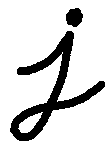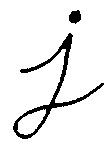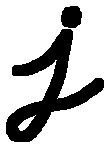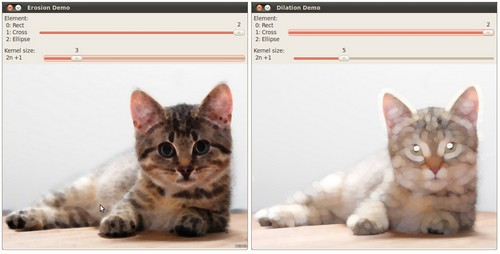OpenCV  3.0.0-rc1 Open Source Computer Vision
Eroding and Dilating

## Goal

In this tutorial you will learn how to:

• Apply two very common morphology operators: Dilation and Erosion. For this purpose, you will use the following OpenCV functions:

## Cool Theory

Note
The explanation below belongs to the book Learning OpenCV by Bradski and Kaehler.

## Morphological Operations

• In short: A set of operations that process images based on shapes. Morphological operations apply a structuring element to an input image and generate an output image.
• The most basic morphological operations are two: Erosion and Dilation. They have a wide array of uses, i.e. :
• Removing noise
• Isolation of individual elements and joining disparate elements in an image.
• Finding of intensity bumps or holes in an image
• We will explain dilation and erosion briefly, using the following image as an example:### Dilation

• This operations consists of convoluting an image $$A$$ with some kernel ( $$B$$), which can have any shape or size, usually a square or circle.
• The kernel $$B$$ has a defined anchor point, usually being the center of the kernel.
• As the kernel $$B$$ is scanned over the image, we compute the maximal pixel value overlapped by $$B$$ and replace the image pixel in the anchor point position with that maximal value. As you can deduce, this maximizing operation causes bright regions within an image to "grow" (therefore the name dilation). Take as an example the image above. Applying dilation we can get:The background (bright) dilates around the black regions of the letter.

### Erosion

• This operation is the sister of dilation. What this does is to compute a local minimum over the area of the kernel.
• As the kernel $$B$$ is scanned over the image, we compute the minimal pixel value overlapped by $$B$$ and replace the image pixel under the anchor point with that minimal value.
• Analagously to the example for dilation, we can apply the erosion operator to the original image (shown above). You can see in the result below that the bright areas of the image (the background, apparently), get thinner, whereas the dark zones (the "writing") gets bigger.## Code

This tutorial code's is shown lines below. You can also download it from here

1
8 #include "opencv2/imgcodecs.hpp"
10 #include <stdlib.h>
11 #include <stdio.h>
12
13 using namespace cv;
14
16 Mat src, erosion_dst, dilation_dst;
17
18 int erosion_elem = 0;
19 int erosion_size = 0;
20 int dilation_elem = 0;
21 int dilation_size = 0;
22 int const max_elem = 2;
23 int const max_kernel_size = 21;
24
26 void Erosion( int, void* );
27 void Dilation( int, void* );
28
32 int main( int, char** argv )
33 {
35  src = imread( argv );
36
37  if( src.empty() )
38  { return -1; }
39
41  namedWindow( "Erosion Demo", WINDOW_AUTOSIZE );
42  namedWindow( "Dilation Demo", WINDOW_AUTOSIZE );
43  moveWindow( "Dilation Demo", src.cols, 0 );
44
46  createTrackbar( "Element:\n 0: Rect \n 1: Cross \n 2: Ellipse", "Erosion Demo",
47  &erosion_elem, max_elem,
48  Erosion );
49
50  createTrackbar( "Kernel size:\n 2n +1", "Erosion Demo",
51  &erosion_size, max_kernel_size,
52  Erosion );
53
55  createTrackbar( "Element:\n 0: Rect \n 1: Cross \n 2: Ellipse", "Dilation Demo",
56  &dilation_elem, max_elem,
57  Dilation );
58
59  createTrackbar( "Kernel size:\n 2n +1", "Dilation Demo",
60  &dilation_size, max_kernel_size,
61  Dilation );
62
64  Erosion( 0, 0 );
65  Dilation( 0, 0 );
66
67  waitKey(0);
68  return 0;
69 }
70
74 void Erosion( int, void* )
75 {
76  int erosion_type = 0;
77  if( erosion_elem == 0 ){ erosion_type = MORPH_RECT; }
78  else if( erosion_elem == 1 ){ erosion_type = MORPH_CROSS; }
79  else if( erosion_elem == 2) { erosion_type = MORPH_ELLIPSE; }
80
81  Mat element = getStructuringElement( erosion_type,
82  Size( 2*erosion_size + 1, 2*erosion_size+1 ),
83  Point( erosion_size, erosion_size ) );
85  erode( src, erosion_dst, element );
86  imshow( "Erosion Demo", erosion_dst );
87 }
88
92 void Dilation( int, void* )
93 {
94  int dilation_type = 0;
95  if( dilation_elem == 0 ){ dilation_type = MORPH_RECT; }
96  else if( dilation_elem == 1 ){ dilation_type = MORPH_CROSS; }
97  else if( dilation_elem == 2) { dilation_type = MORPH_ELLIPSE; }
98
99  Mat element = getStructuringElement( dilation_type,
100  Size( 2*dilation_size + 1, 2*dilation_size+1 ),
101  Point( dilation_size, dilation_size ) );
103  dilate( src, dilation_dst, element );
104  imshow( "Dilation Demo", dilation_dst );
105 }
Mat getStructuringElement(int shape, Size ksize, Point anchor=Point(-1,-1))
Returns a structuring element of the specified size and shape for morphological operations.
Definition: imgproc.hpp:235
Loads an image from a file.
void erode(InputArray src, OutputArray dst, InputArray kernel, Point anchor=Point(-1,-1), int iterations=1, int borderType=BORDER_CONSTANT, const Scalar &borderValue=morphologyDefaultBorderValue())
Erodes an image by using a specific structuring element.
void imshow(const String &winname, InputArray mat)
Displays an image in the specified window.
int createTrackbar(const String &trackbarname, const String &winname, int *value, int count, TrackbarCallback onChange=0, void *userdata=0)
Creates a trackbar and attaches it to the specified window.
void dilate(InputArray src, OutputArray dst, InputArray kernel, Point anchor=Point(-1,-1), int iterations=1, int borderType=BORDER_CONSTANT, const Scalar &borderValue=morphologyDefaultBorderValue())
Dilates an image by using a specific structuring element.
void namedWindow(const String &winname, int flags=WINDOW_AUTOSIZE)
Creates a window.
Size2i Size
Definition: types.hpp:308
a rectangular structuring element:
Definition: imgproc.hpp:234
Definition: highgui.hpp:138
void moveWindow(const String &winname, int x, int y)
Moves window to the specified position.
Definition: imgproc.hpp:237
int main(int argc, const char *argv[])
Definition: facerec_demo.cpp:67
n-dimensional dense array class
Definition: mat.hpp:726
Point2i Point
Definition: types.hpp:181
int waitKey(int delay=0)
Waits for a pressed key.

## Explanation

1. Most of the stuff shown is known by you (if you have any doubt, please refer to the tutorials in previous sections). Let's check the general structure of the program:

• Load an image (can be RGB or grayscale)
• Create two windows (one for dilation output, the other for erosion)
• Create a set of 02 Trackbars for each operation:
• The first trackbar "Element" returns either erosion_elem or dilation_elem
• The second trackbar "Kernel size" return erosion_size or dilation_size for the corresponding operation.
• Every time we move any slider, the user's function Erosion or Dilation will be called and it will update the output image based on the current trackbar values.

Let's analyze these two functions:

2. erosion:
/* @function Erosion */
void Erosion( int, void* )
{
int erosion_type;
if( erosion_elem == 0 ){ erosion_type = MORPH_RECT; }
else if( erosion_elem == 1 ){ erosion_type = MORPH_CROSS; }
else if( erosion_elem == 2) { erosion_type = MORPH_ELLIPSE; }
Mat element = getStructuringElement( erosion_type,
Size( 2*erosion_size + 1, 2*erosion_size+1 ),
Point( erosion_size, erosion_size ) );
erode( src, erosion_dst, element );
imshow( "Erosion Demo", erosion_dst );
}
• The function that performs the erosion operation is cv::erode . As we can see, it receives three arguments:
• src: The source image
• erosion_dst: The output image
• element: This is the kernel we will use to perform the operation. If we do not specify, the default is a simple 3x3 matrix. Otherwise, we can specify its shape. For this, we need to use the function cv::getStructuringElement :

Mat element = getStructuringElement( erosion_type,
Size( 2*erosion_size + 1, 2*erosion_size+1 ),
Point( erosion_size, erosion_size ) );

We can choose any of three shapes for our kernel:

• Rectangular box: MORPH_RECT
• Cross: MORPH_CROSS
• Ellipse: MORPH_ELLIPSE

Then, we just have to specify the size of our kernel and the anchor point. If not specified, it is assumed to be in the center.

• That is all. We are ready to perform the erosion of our image.
Note
Additionally, there is another parameter that allows you to perform multiple erosions (iterations) at once. We are not using it in this simple tutorial, though. You can check out the Reference for more details.
3. dilation:

The code is below. As you can see, it is completely similar to the snippet of code for erosion. Here we also have the option of defining our kernel, its anchor point and the size of the operator to be used.

/* @function Dilation */
void Dilation( int, void* )
{
int dilation_type;
if( dilation_elem == 0 ){ dilation_type = MORPH_RECT; }
else if( dilation_elem == 1 ){ dilation_type = MORPH_CROSS; }
else if( dilation_elem == 2) { dilation_type = MORPH_ELLIPSE; }
Mat element = getStructuringElement( dilation_type,
Size( 2*dilation_size + 1, 2*dilation_size+1 ),
Point( dilation_size, dilation_size ) );
dilate( src, dilation_dst, element );
imshow( "Dilation Demo", dilation_dst );
}

## Results

Compile the code above and execute it with an image as argument. For instance, using this image:We get the results below. Varying the indices in the Trackbars give different output images, naturally. Try them out! You can even try to add a third Trackbar to control the number of iterations.